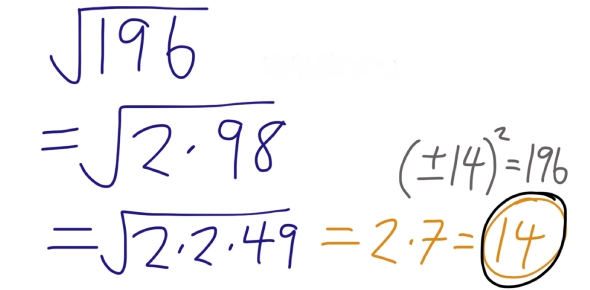# Square Roots Of Perfect And Nonperfect Squaress

6 Questions | Total Attempts: 376SettingsMathematics is an interesting subject and is one that you can never miss to take in any level of study. The quiz below is based on the square roots of perfect and non-perfect squares. Take the quiz below and gauge your knowledge.

• 1.
What is the square root of 6?
• 2.
What is the square root of 75?
• 3.
What is the square root of 49?
• 4.
What is the square root of 17?
• 5.
What is the square root of 42?
• 6.
What is the square root of 144?
Related TopicsBack to top